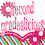## Thursday, April 11, 2013

### Is it Equal??

One of my newest math products is an equal/not equal sort.  I started with just addition.  I created a bunch of equal equations and not equal equations.  Then I decided it was time to introduce my firsties to the fact that sometimes the equation can be written with the sum first (before the equal sign).  So, I included some of these equations into my sorts.  Then, I branched out and created one for subtraction equations and one in which there was a mix of addition and subtraction.
You can get all three sorts in this pack here, or check out my store to get them each individually!

When my firsties tried this for the first time, I was amazed.  It really seemed to split my class into two groups: the kids that have a full grasp of addition and subtraction and those that still were just not getting it.  After quite a bit of practice, though, I am proud to say that this activity has really helped my kids to have a deeper understanding of these skills!  I am so proud!

#### 1 comment:

1.Isn't it great when they finally "get" it?!